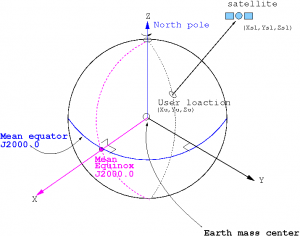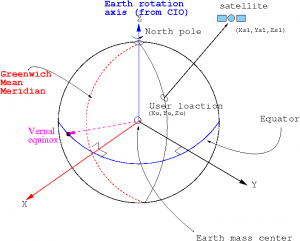If you wish to contribute or participate in the discussions about articles you are invited to contact the Editor

# Reference Systems and FramesFundamentals
Title Reference Systems and Frames
Author(s) J. Sanz Subirana, J.M. Juan Zornoza and M. Hernández-Pajares, Technical University of Catalonia, Spain.
Level Basic
Year of Publication 2011

Satellite coordinates and user receivers must be expressed in a well defined reference system. Thence, an accurate definition and determination of such systems is essential to assure a precise positioning in GNSS. Two of the main reference systems used in satellite navigation are introduced bellow: The Conventional Celestial Reference System (also named Conventional Inertial System, CIS) and the Conventional Terrestrial Reference System (also named Coordinated Terrestrial System, CTS).

• Conventional Celestial Reference System (CRS) This is a quasi-inertial reference system[footnotes 1]. It has its origin at the earth's centre of mass. X-axis points in the direction of the mean equinox at J2000.0 epoch, Z-axis is orthogonal to the plane defined by the mean equator at J2000.0 epoch (fundamental plane) and Y-axis is orthogonal to the former ones, so the system is directly (right handed) oriented. The practical implementation is called (conventional) Celestial Reference Frame (CRF)[footnotes 2] and it is determined from a set of precise coordinates of extragalactic radio sources (i.e., it is fixed with respect to distant objects of the universe). The mean equator and equinox J2000.0 were defined by International Astronomical Union (IAU) agreements in 1976, with 1980 nutation series (Seildelmann, 1982 and Kaplan, 1981), which are valid analytic expressions for long time intervals (the former reference epoch was 1950.0).
• Conventional Terrestrial Reference System (TRS) This is a reference system co-rotating with the earth in its diurnal rotation, also called Earth-Centred, Earth-Fixed (ECEF). Its definition involves a mathematical model for a physical earth in which point positions are expressed and have small temporal variations due to geophysical effects (plate motion, earth tides, etc.). The TRS has its origin in the earth's centre of mass. Z-axis is identical to the direction of the earth's rotation axis defined by the Conventional Terrestrial Pole (CTP), X-axis is defined as the intersection of the orthogonal plane to Z-axis (fundamental plane) and Greenwich mean meridian, and Y-axis is orthogonal to both of them, making the system directly oriented. The realization of this system is named (conventional) Terrestrial Reference Frame (TRF) and it is carried out through the coordinates of a set of points on the earth serving as reference points[footnotes 3]. An example of TRF is the International Terrestrial Reference Frame (ITRF) introduced by the International Earth Rotation and Reference Systems Service (IERS), which is updated every year (ITRF98, ITRF99, etc.). Other terrestrial reference frames are the World Geodetic System 84 (WGS-84), which is applied for GPS, the Parametry Zemli 1990 (Parameters of the Earth 1990) (PZ-90) for GLONASS, or the Galileo Terrestrial Reference Frame (GTRF) for Galileo system.Figure 1: Celestial Reference System (CRS)Figure 2: Terrestrial Reference System (TRS)